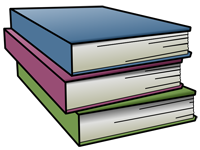• Overview of Grade 6 Math

Sixth grade students will learn foundational algebraic, geometric, and statistical concepts. Students use variables extensively with expressions, equations and formulas, and develop the understanding of dependent and independent variables for graphing. Mathematics practices of perseverance, reasoning, justifying, modeling, using appropriate tools, attending to precision, looking for and using patterns are strongly applied in sixth grade. Geometric concepts of area, volume, and surface areas are related to real-world problem solving. Students apply measures of center and measures of variability for the first time during sixth grade.